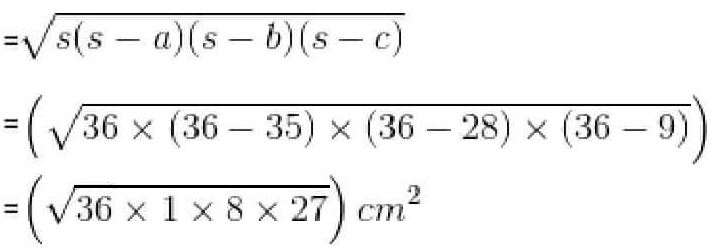Guru

# A floral design on a floor is made up of 16 tiles which are triangular, the sides of the triangle being 9 cm, 28 cm and 35 cm (see Fig. 12.18). Find the cost of polishing the tiles at the rate of 50p per cm2 . Q.8

• 0

what is the tricky way for solving the question of class 9th ncert math of Heron’s Formula chapter of ncert of exercise 12.2  of math give me the best and simple way for solving this question in easy and simple way of question no.8 A floral design on a floor is made up of 16 tiles which are triangular, the sides of the triangle being 9 cm, 28 cm and 35 cm (see Fig. 12.18). Find the cost of polishing the tiles at the rate of 50p per cm2 .

Share

1. The semi perimeter of the each triangular shape = (28+9+35)/2 cm = 36 cm

By using Heron’s formula,

The area of each triangular shape will be= 36√6 cm2 = 88.2 cm2

Now, the total area of 16 tiles = 16×88.2 cm2 = 1411.2 cm2

It is given that the polishing cost of tiles = 50 paise/cm2

∴ The total polishing cost of the tiles = Rs. (1411.2×0.5) = Rs. 705.6

• 0